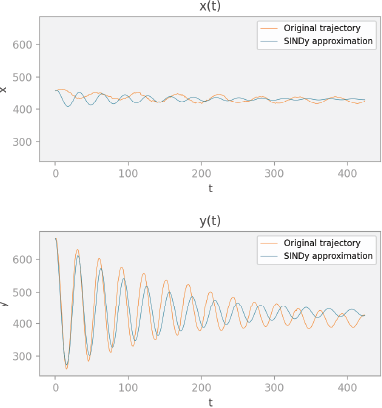Hi, I’m Bea Stollnitz, and I’m a principal developer advocate at Microsoft, focusing on Azure ML.

All code in this blog is made available under the MIT license.

• # Azure ML

• ## Creating managed online endpoints in Azure ML

In this post, you’ll learn how to create endpoints on Azure that enable real-time predictions using your custom model. I assume familiarity with machine learning concepts, such as training and prediction, but no knowledge of Azure.• ## Creating batch endpoints in Azure ML

In this post, you’ll learn how to create endpoints on Azure that enable asynchronous batch predictions using your custom model. I assume familiarity with machine learning concepts, such as training and prediction, but no knowledge of Azure.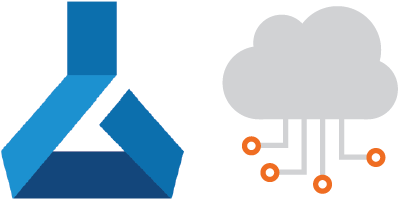• ## Choosing the compute for Azure ML resources

When creating Azure ML resources you often have to decide which compute to use to run your code. This post will help you understand how to make the right choice for your scenario. I assume some familiarity with Azure ML in order to follow along.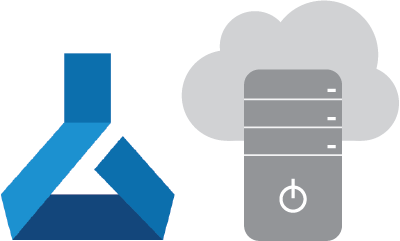• ## Choosing the environment for Azure ML resources

In this post, you will learn about the different types of environments you can choose from when creating a new Azure ML resource. I assume some familiarity with Azure ML in order to follow along.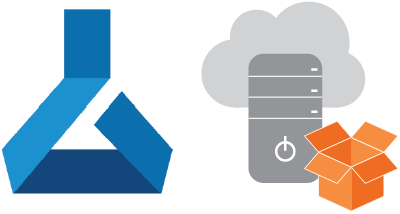• # Development tools

• ## Configuring Azure ML projects to run on GitHub Codespaces

In this post, you’ll learn how to run your Azure ML projects on GitHub Codespaces, a new cloud-based development environment that runs your code in a container. I’ll also cover several best practices for configuring VS Code settings for machine learning projects. I assume some familiarity with Git, GitHub, VS Code, Python, Conda, and Azure ML.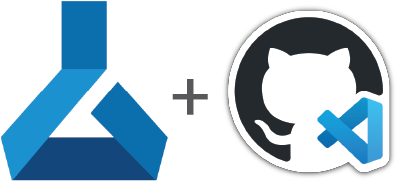• ## Configuring your terminal on GitHub Codespaces

In this post, you’ll learn how to configure your terminal on GitHub Codespaces, ensuring that your remote environment feels as familiar as your local one. The content of this post is useful to anyone using VS Code and GitHub Codespaces, whether you’re working on machine learning projects or not.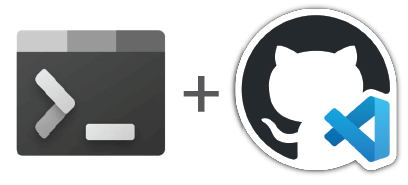• # ML frameworks and libraries

• ## Introduction to PyTorch

This post introduces PyTorch concepts through the creation of a basic neural network using the Fashion MNIST dataset as a data source. I assume that you have a basic conceptual understanding of neural networks, and that you’re comfortable with Python, but I assume no knowledge of PyTorch.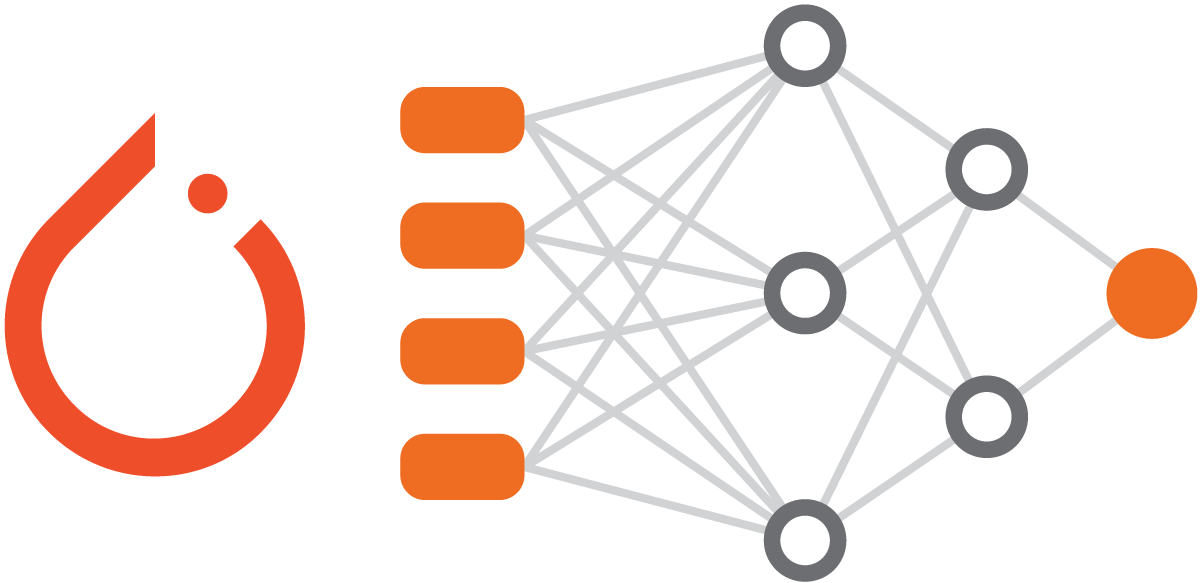• ## Introduction to TensorFlow using Keras

This post provides all the concepts and practical knowledge you need to get started with TensorFlow. We’ll explore Keras, a high-level API released as part of TensorFlow, and we’ll use it to build a basic neural network using the Fashion MNIST dataset as a data source. I assume that you have a basic conceptual understanding of neural networks, and that you’re comfortable with Python, but I assume no knowledge of TensorFlow or Keras.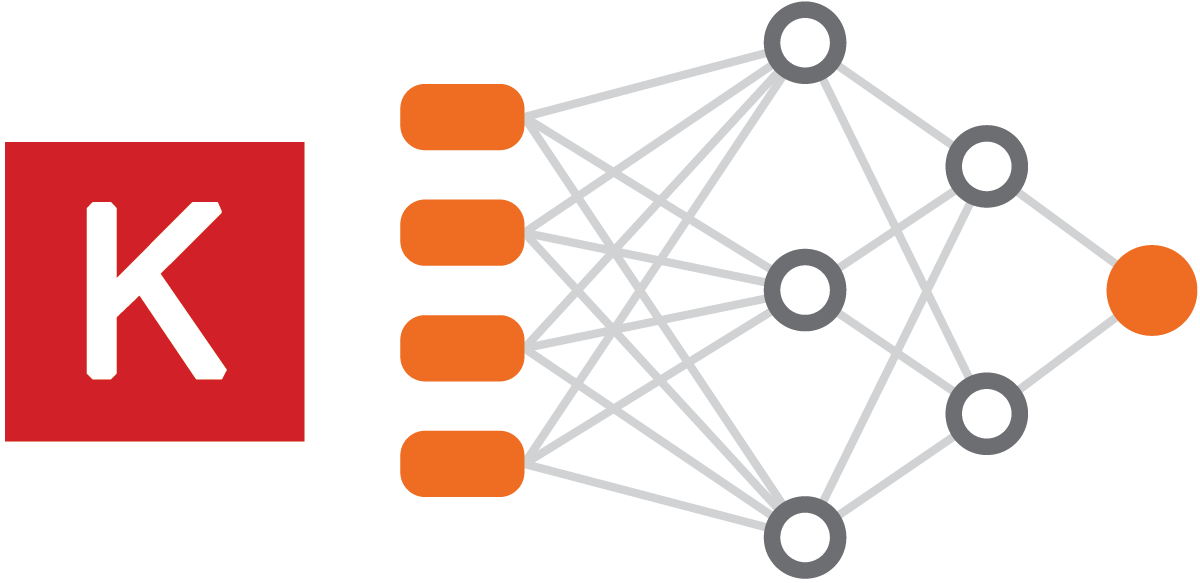• ## Going beyond Keras - customizing with TensorFlow

In this blog post, we’ll re-implement parts of the code from my earlier Keras post, but this time we’ll use lower-level TensorFlow concepts. I assume that you completed my tutorial on Keras or that you have a solid knowledge of Keras, but I assume no knowledge of TensorFlow.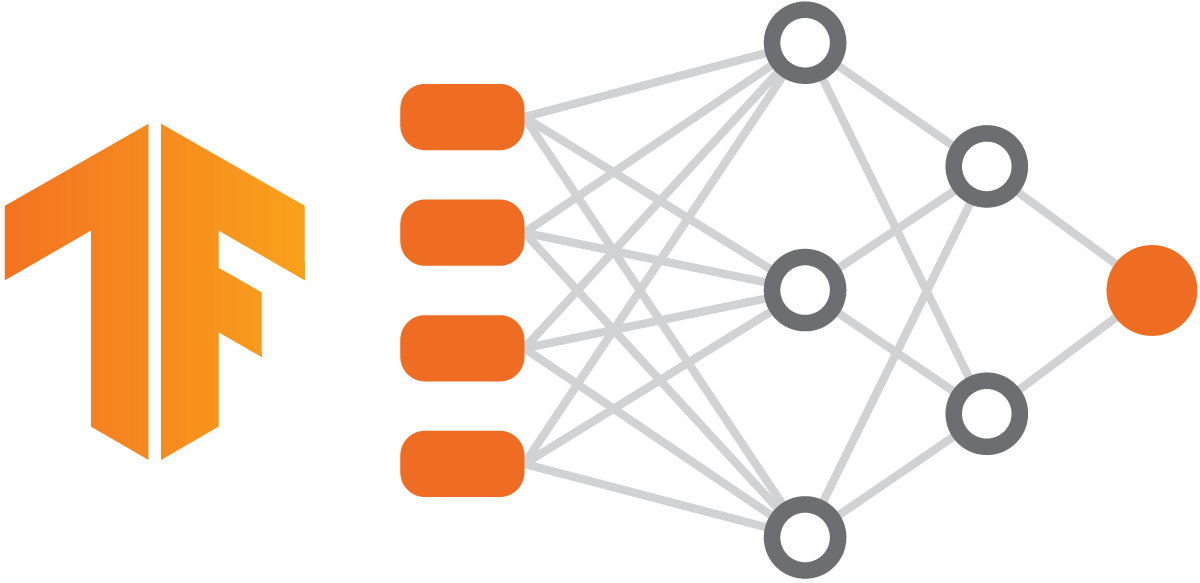• ## Comparing PyTorch and TensorFlow implementations

How do PyTorch code and TensorFlow code compare? Maybe you’re in the beginning phases of your machine learning journey and deciding which framework to embrace, or maybe you’re an experienced ML practicioner considering a change of framework. Either way, you’re in the right place. Drawing from my previous posts, I’ll compare the PyTorch and TensorFlow versions of the code used to classify images in the Fashion MNIST dataset.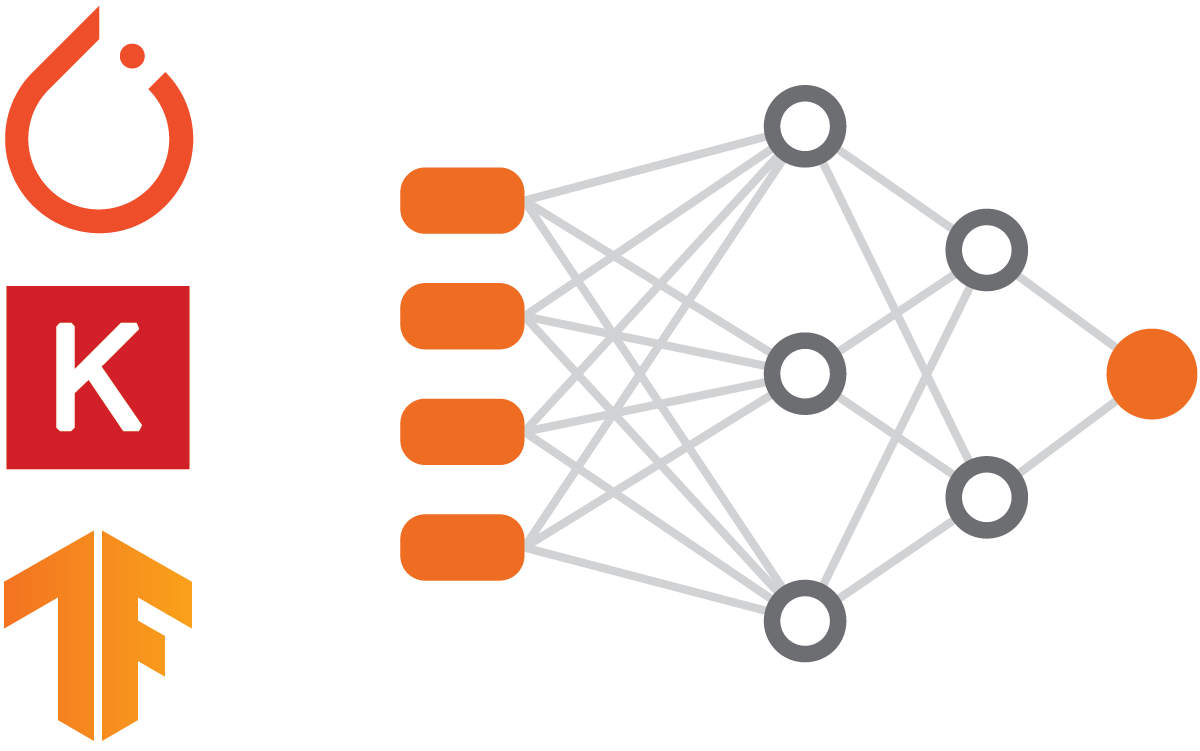• # ML techniques

• ## Creating spectrograms and scaleograms for signal classification

In this post, I’ll explain how to convert time-series signals into spectrograms and scaleograms. In a future post, we’ll use the images created here to classify the signals. I assume that you have basic math skills and are familiar with basic machine learning concepts.• ## Discovering equations from data using SINDy

In this post, I’ll discuss the 2016 paper “Discovering Governing Equations from Data by Sparse Identification of Nonlinear Dynamical Systems” by Brunton et al. I’ll explain the main concepts of the paper in an accessible way, and I’ll show how we can use its novel approach to discover the Lorenz system of equations from data. I assume basic familiarity with ordinary differential equations and dynamical systems.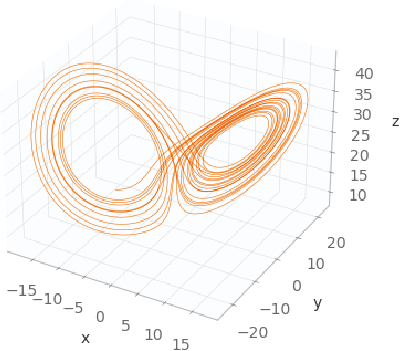• ## Using PySINDy to discover equations from experimental data

In this post, I will use the PySINDy Python package to discover a system of ordinary differential equations that best represents my experimental data. I assume that you read my post “Discovering equations from data using SINDy,” and that you have basic familiarity with ordinary differential equations and dynamical systems.